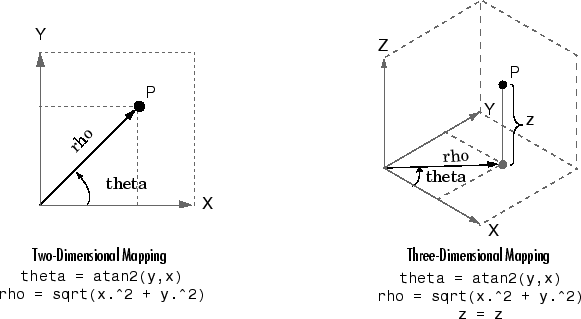# cart2pol

## 语法

``[theta,rho] = cart2pol(x,y)``
``[theta,rho,z] = cart2pol(x,y,z)``

## 说明

``[theta,rho] = cart2pol(x,y)` 可将二维笛卡尔坐标数组 `x` 和 `y` 的对应元素变换为极坐标 `theta` 和 `rho`。`

``[theta,rho,z] = cart2pol(x,y,z)` 可将三维笛卡尔坐标数组 `x`、`y` 和 `z` 变换为柱坐标 `theta`、`rho` 和 `z`。`

## 示例

`x = [5 3.5355 0 -10]`
```x = 1×4 5.0000 3.5355 0 -10.0000 ```
`y = [0 3.5355 10 0]`
```y = 1×4 0 3.5355 10.0000 0 ```
`[theta,rho] = cart2pol(x,y)`
```theta = 1×4 0 0.7854 1.5708 3.1416 ```
```rho = 1×4 5.0000 5.0000 10.0000 10.0000 ```

`x = [1 2.1213 0 -5]'`
```x = 4×1 1.0000 2.1213 0 -5.0000 ```
`y = [0 2.1213 4 0]'`
```y = 4×1 0 2.1213 4.0000 0 ```
`z = [7 8 9 10]'`
```z = 4×1 7 8 9 10 ```
`[theta,rho,z] = cart2pol(x,y,z)`
```theta = 4×1 0 0.7854 1.5708 3.1416 ```
```rho = 4×1 1.0000 3.0000 4.0000 5.0000 ```
```z = 4×1 7 8 9 10 ```

## 算法